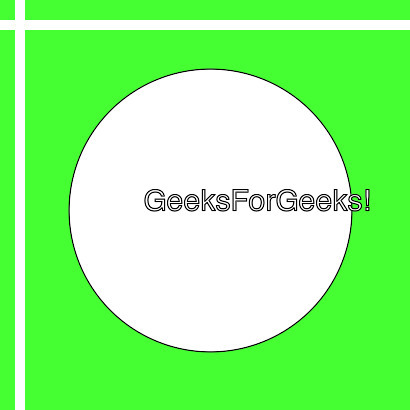Related Articles
Wand splice() function – Python
• Last Updated : 04 May, 2020

The splice() function is an inbuilt function in the Python Wand ImageMagick library which is used to partition the image by splicing a width x height rectangle at (x, y) offset coordinate. The space inserted will be replaced by the available background color.

Syntax:

`splice(width, height, x, y)`

Parameters: This function accepts four parameters as mentioned above and defined below:

• width: This parameter stores the width of the rectangle.
• height: This parameter stores the height of the recangle.
• x: This parameter stores the offset on the x-axis.
• y: This parameter stores the offset on the y-axis.

Return Value: This function returns the Wand ImageMagick object.

Original Image:Example 1:

 `# Import library from Image ``from` `wand.image ``import` `Image`` ` `# Import the image``with Image(filename ``=``'../geeksforgeeks.png'``) as image:``    ``# Clone the image in order to process``    ``with image.clone() as splice:``        ``# Invoke splice function with height as 20, width as 20, x as 10, y as 10``        ``splice.splice(``20``, ``20``, ``10``, ``10``)``        ``# Save the image``        ``splice.save(filename ``=``'splice1.jpg'``)`

Output:Example 2:

 `# Import libraries from the wand  ``from` `wand.image ``import` `Image``from` `wand.drawing ``import` `Drawing``from` `wand.color ``import` `Color`` ` `with Drawing() as draw:``    ``# Set Stroke color the circle to black``    ``draw.stroke_color ``=` `Color(``'black'``)``    ``# Set Width of the circlw to 2 ``    ``draw.stroke_width ``=` `1``    ``# Set the fill color to 'White (# FFFFFF)'``    ``draw.fill_color ``=` `Color(``'white'``)`` ` `    ``# Invoke Circle function with center at 50, 50 and radius 25``    ``draw.circle((``200``, ``200``), ``# Center point``                ``(``100``, ``100``)) ``# Perimeter point``    ``# Set the font style``    ``draw.font ``=` `'../Helvetica.ttf'``    ``# Set the font size ``    ``draw.font_size ``=` `30``     ` `    ``with Image(width ``=` `400``, height ``=` `400``, background ``=` `Color(``'# 45ff33'``)) as pic:``        ``# Set the text and its location``        ``draw.text(``int``(pic.width ``/` `3``), ``int``(pic.height ``/` `2``), ``'GeeksForGeeks !'``)``        ``# Draw the picture``        ``draw(pic)``        ``# Invoke splice function with height as 10, width as 10, x as 15, y as 20``        ``pic.splice(``10``, ``10``, ``15``, ``20``)``        ``# Save the image ``        ``pic.save(filename ``=``'splice2.jpg'``)`

Output:Attention geek! Strengthen your foundations with the Python Programming Foundation Course and learn the basics.

To begin with, your interview preparations Enhance your Data Structures concepts with the Python DS Course.

My Personal Notes arrow_drop_up